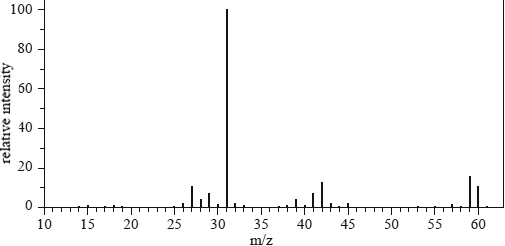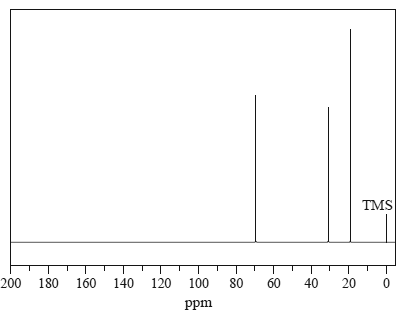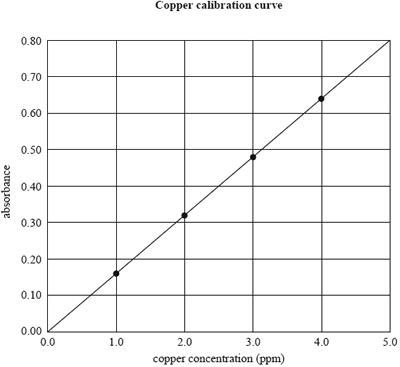Spectroscopy (2014 VCE) 1) The mass spectrum of alkanol T is provided below.What is alkanol T? A. butan-1-ol B. ethanol C. methanol D. propan-1-ol Solution2) The 13C NMR spectrum below corresponds to which one of the following compounds? A. propane B. 2-methylpropane C. 2-methylpropan-1-ol D. 2-methylpropan-2-olSolution 3) An atomic absorption spectrometer can be used to determine the level of copper in soils. The calibration curve below plots the absorbance of four standard copper solutions against the concentration of copper ions in ppm. The concentrations of copper ions in the standard solutions were 1.0, 2.0, 3.0 and 4.0 mg L–1. (1 mg L–1 = 1 ppm)The concentration of copper in a test solution can be determined most accurately from the calibration curve if it is between A. 0.0 ppm and 5.0 ppm. B. 0.0 ppm and 4.0 ppm. C. 1.0 ppm and 4.0 ppm. D. 1.0 ppm and 5.0 ppm. Solutionb) If the test solution gave an absorbance reading of 0.40, what would be the concentration of copper ions in the solution in mol L–1? A. 2.5 B. 3.9 × 10–2 C. 3.9 × 10–5 D. 2.5 × 10–6 Solution4) To determine the amount of phosphate in a sample of polluted water, a coloured solution is produced by adding excess molybdovanadate reagent to the water sample. Which technique would be used to determine the concentration of phosphate in the water? A. atomic absorption spectroscopy B. gas chromatography C. high-performance liquid chromatography D. ultraviolet – visible spectroscopy   Solution5) A small organic molecule has the molecular formula of the form CxHyO2Cl. A pH probe was inserted into a dilute aqueous solution of this compound and the pH was 4.5. The mass spectrum, infrared spectrum, 1H NMR spectrum and 13C NMR spectrum of this compound are provided a) On the infrared spectrum,identify the wavelength that corresponds to the presence of two functional groups in this compound. Identify the functional groups. Note: The peak due to the C-Cl stretch has been labelled. Solutionb) Use the data provided to determine the values of x and y in CxHyO2Cl.. Solutionc) What specific information about the structure of the compound is provided by the splitting pattern in the 1H NMR spectrum? Solutiond) Draw the complete molecular structure for this molecule. Solutione) Give a reason why the mass spectrum shows two molecular ion peaks at m/z = 108 and 110, rather than just one. Solution